## Example Questions

← Previous 1 3

### Example Question #1 : How To Find The Length Of A Line With Distance Formula

What is the distance between (1, 4) and (5, 1)?

9

7

4

3

5

5

Explanation:

Let P1 = (1, 4) and P2 = (5, 1)

Substitute these values into the distance formula: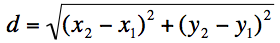The distance formula is an application of the Pythagorean Theorem:  a2 + b2 = c2

### Example Question #1 : How To Find The Length Of A Line With Distance Formula

What is the distance of the line drawn between points (–1,–2) and (–9,4)?

4

√5

6

16

10

10

Explanation:

The answer is 10. Use the distance formula between 2 points, or draw a right triangle with legs length 6 and 8 and use the Pythagorean Theorem.

### Example Question #31 : Coordinate Geometry

What is the distance between two points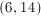and?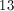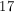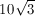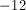Explanation:

To find the distance between two points such as these, plot them on a graph.

Then, find the distance between theunits of the points, which is 12, and the distance between the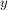points, which is 5. Therepresents the horizontal leg of a right triangle and therepresents the vertial leg of a right triangle. In this case, we have a 5,12,13 right triangle, but the Pythagorean Theorem can be used as well.

### Example Question #1 : Distance Formula

What is the distance between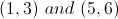?Explanation:

Let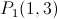and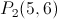and use the distance formula: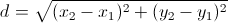### Example Question #1 : How To Find The Length Of A Line With Distance Formula

What is the distance between the point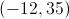and the origin?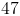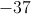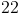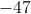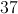Explanation:

The distance between 2 points is found using the distance or Pythagorean Theorem.  Because values are squared in the formula, distance can never be a negative value.

### Example Question #2 : How To Find The Length Of A Line With Distance Formula

Bill gets in his car and drives north for 30 miles at 40 mph.  He then turns west and drives 40 mph for 40 minutes.  Finally, he goes directly northeast 40 miles in 25 minutes.

Using the total distance traveled as a straight line ("as the crow flies") and the time spent traveling, which of the following is closest to Bill's average speed?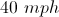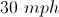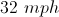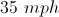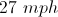Explanation:

Each part of the problem gives you 2 out of the 3 pieces of the rate/time/distance relationship, thus allowing you to find the third (if needed) by using the equation: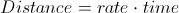The problem is otherwise an application of geometry and the distance formula.  We need to find the distance between 2 points, but we need to go step-by-step to find out where the final point is.  The first two steps are relatively easy to follow.  He travels 30 miles north.  Now he turns west and travels for 40 minutes at 40 mph.  This isof an hour, so we have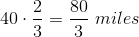.  Thus if we are looking at standard Cartesian coordinates (starting at the origin), we are now at the point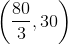.

We are now on the last step: 40 miles northeast.  We need to decipher this into- and- coordinate changes.  To do this, we think of a triangle.  Because we are moving directly northeast, this is a 45 degree angle to the horizontal.  We can thus imagine a 45-45-90 triangle with a hypotenuse of 40.  Now using the relationships on triangles we have: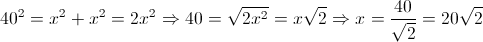So the final step moves us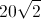up and to the right.  Moving this way from the pointleaves us at: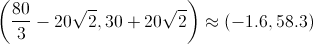Using the distance formula for this point from the origin gives us a distance of ~58 miles.

Now for the time.  We traveled 30 miles at 40 mph.  This means we traveled for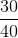hours or 45 minutes.

We then traveled 40 mph for 40 minutes. Increasing our total time traveled to 85 minutes.

Finally, we traveled 40 miles in 25 minutes, leaving our total time traveled at 110 minutes.  Returning to hours, we have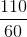hours or 1.83 hours.

Our final average speed traveled is then: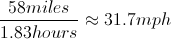The closest answer to this value is 32 mph.

### Example Question #1 : How To Find The Length Of A Line With Distance Formula

Steven draws a line that is 13 units long. If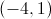is one endpoint of the line, which of the following might be the other endpoint?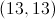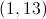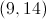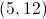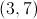Explanation:

The distance formula is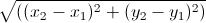.

Plug inwith each of the answer choices and solve.

Plug in: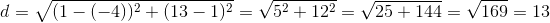This is therefore the correct answer choice.

### Example Question #2 : Distance Formula

What is the distance between the points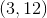and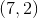?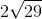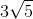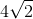Explanation:

Plug the points into the distance formula and simplify:

distance2 = (x2 – x1)2 + (y2 – y1)2 = (7 – 3)2 + (2 – 12)2 = 42 + 102 = 116

distance = √116 = √(4 * 29) = 2√29

### Example Question #1 : Distance Formula

What is the distance between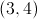and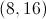?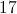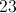Explanation:

The formula for the distance between two points is.

Plug in the points: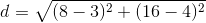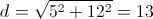### Example Question #1 : Distance Formula

Suppose a line is connected from two points.  What is the distance of the line if the line is connected from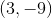to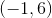?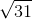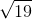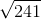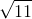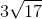Explanation:

Write the distance formula and substitute the values into the formula.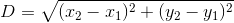Let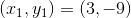and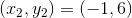.

Plugging in our coordinates into the distance formula we get the following.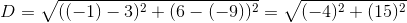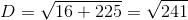← Previous 1 3

### All SAT Math Resources